# Common fractions and decimals#### Everything You Need in One Place

Homework problems? Exam preparation? Trying to grasp a concept or just brushing up the basics? Our extensive help & practice library have got you covered.#### Learn and Practice With Ease

Our proven video lessons ease you through problems quickly, and you get tonnes of friendly practice on questions that trip students up on tests and finals.#### Instant and Unlimited Help

Our personalized learning platform enables you to instantly find the exact walkthrough to your specific type of question. Activate unlimited help now!

0/5
##### Intros
###### Lessons
1. Introduction to Common Fractions and Decimals:
2. How are decimals related to fractions?
3. How to convert decimal tenths into decimal fractions ($\large \frac{1}{10}$)
4. How to convert decimal hundredths into decimal fractions ($\large \frac{1}{100}$)
5. How to convert between decimals and common fraction halves ($\large \frac{1}{2}$)
6. How to convert between decimals and common fraction quarters ($\large \frac{1}{4}$)
0/13
##### Examples
###### Lessons
1. Writing common fractions as decimals
Write the following fractions as decimals
1. Unit fractions:
2. Decimal fractions:
3. Common fractions related to $\large \frac{1}{2}$ or $\large \frac{1}{4}$:
2. Fraction and figure equivalents for decimals
Complete the table for the equivalent decimal, fraction, and shaded in figure:
1.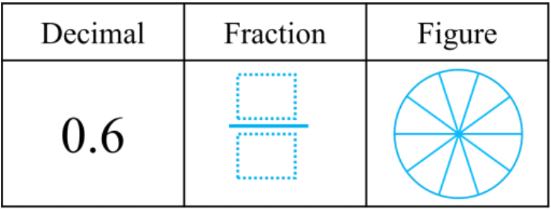2.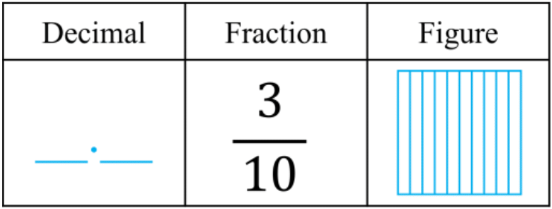3.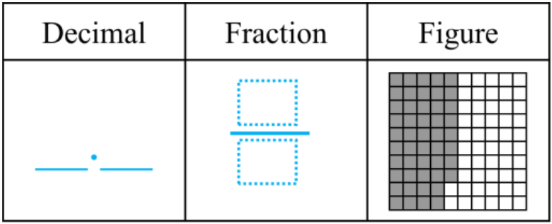4.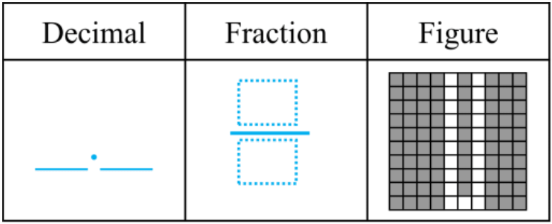3. Fraction and figure equivalents for decimals
Complete the table for the equivalent decimal, fraction, and shaded in figure:
1. 0.28 = $\large \frac{}{100}$ = $\large \frac{20}{100}$ + $\large \frac{}{100}$ = $\large \frac{}{10}$ + $\large \frac{}{100}$
2. 0.51 = $\large \frac{}{100}$ = $\large \frac{50}{100}$ + $\large \frac{}{100}$ = $\large \frac{}{10}$ + $\large \frac{}{100}$
3. 0.73 = $\large \frac{?}{?}$ = $\large \frac{}{100}$ + $\large \frac{}{100}$ = $\large \frac{}{10}$ + $\large \frac{}{100}$
4. 0.49 = $\large \frac{?}{?}$ = $\large \frac{?}{?}$ + $\large \frac{?}{?}$ = $\large \frac{?}{?}$ + $\large \frac{?}{?}$
4. Common fractions and decimal number lines
Using common fractions and decimals:

A:$\large \frac{25}{100} \qquad \;$ B:$\large \frac{7}{10} \qquad \;$ C:$\large \frac{90}{100} \qquad \;$ D:$\large \frac{5}{10} \qquad \;$ A:$\large \frac{75}{100} \qquad$
1. Draw each fraction as a point on the number line. Label each point with its corresponding letter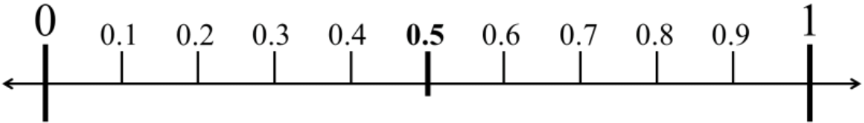2. Which fractions can be rewritten in lowest forms as common fraction halves and quarters?
0%
##### Practice
###### Free to Join!
StudyPug is a learning help platform covering math and science from grade 4 all the way to second year university. Our video tutorials, unlimited practice problems, and step-by-step explanations provide you or your child with all the help you need to master concepts. On top of that, it's fun - with achievements, customizable avatars, and awards to keep you motivated.
• #### Easily See Your ProgressWe track the progress you've made on a topic so you know what you've done. From the course view you can easily see what topics have what and the progress you've made on them. Fill the rings to completely master that section or mouse over the icon to see more details.
• #### Make Use of Our Learning Aids###### Practice Accuracy

Get quick access to the topic you're currently learning.

See how well your practice sessions are going over time.

Stay on track with our daily recommendations.

• #### Earn Achievements as You LearnMake the most of your time as you use StudyPug to help you achieve your goals. Earn fun little badges the more you watch, practice, and use our service.
• #### Create and Customize Your AvatarPlay with our fun little avatar builder to create and customize your own avatar on StudyPug. Choose your face, eye colour, hair colour and style, and background. Unlock more options the more you use StudyPug.
###### Topic Notes

In this lesson, we will learn:

• How to convert between decimals and decimal fractions (tenths and hundredths)
• How to convert between decimals and unit fractions
• How to convert between decimals and fraction halves and quarters

Notes:

• Decimals can be converted into fractions and vice versa
• Recall that place values are related to their neighbors by a factor of 10

•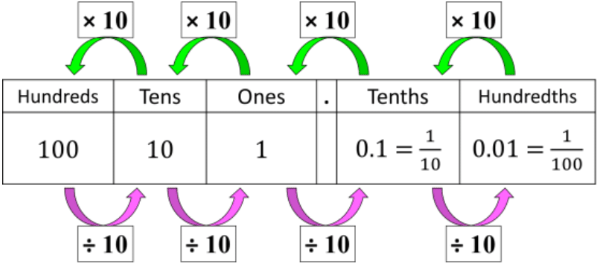• Decimals can be easily converted into decimal fractions
• Decimal fractions have denominators that are powers of 10
• Decimal tenths are fractions out of 10
• Ex. 0.3 = $\large \frac{3}{10}$; 3 tenths = 3 out of 10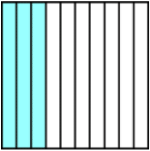• Decimal hundredths are fractions out of 100
• Ex. 0.22 = $\large \frac{22}{100}$; 22 hundredths = 22 out of 100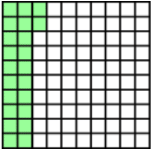• Decimal hundredths can also be expressed as a sum of fractions, because of equivalent decimals (i.e. 0.2 = 0.20)
• 0.22 = $\large \frac{22}{100}$ = $\large \frac{20}{100}$ + $\large \frac{2}{100}$ = $\large \frac{2}{10}$ + $\large \frac{2}{100}$

• Two type of common fractions have a denominator of 2 or 4

 Type Fraction Decimal Value "half" $\frac{1}{2}$ = $\frac{50}{100}$ = $\frac{5}{10}$ 0.5 "quarter" $\frac{1}{4}$ = $\frac{25}{100}$ 0.25